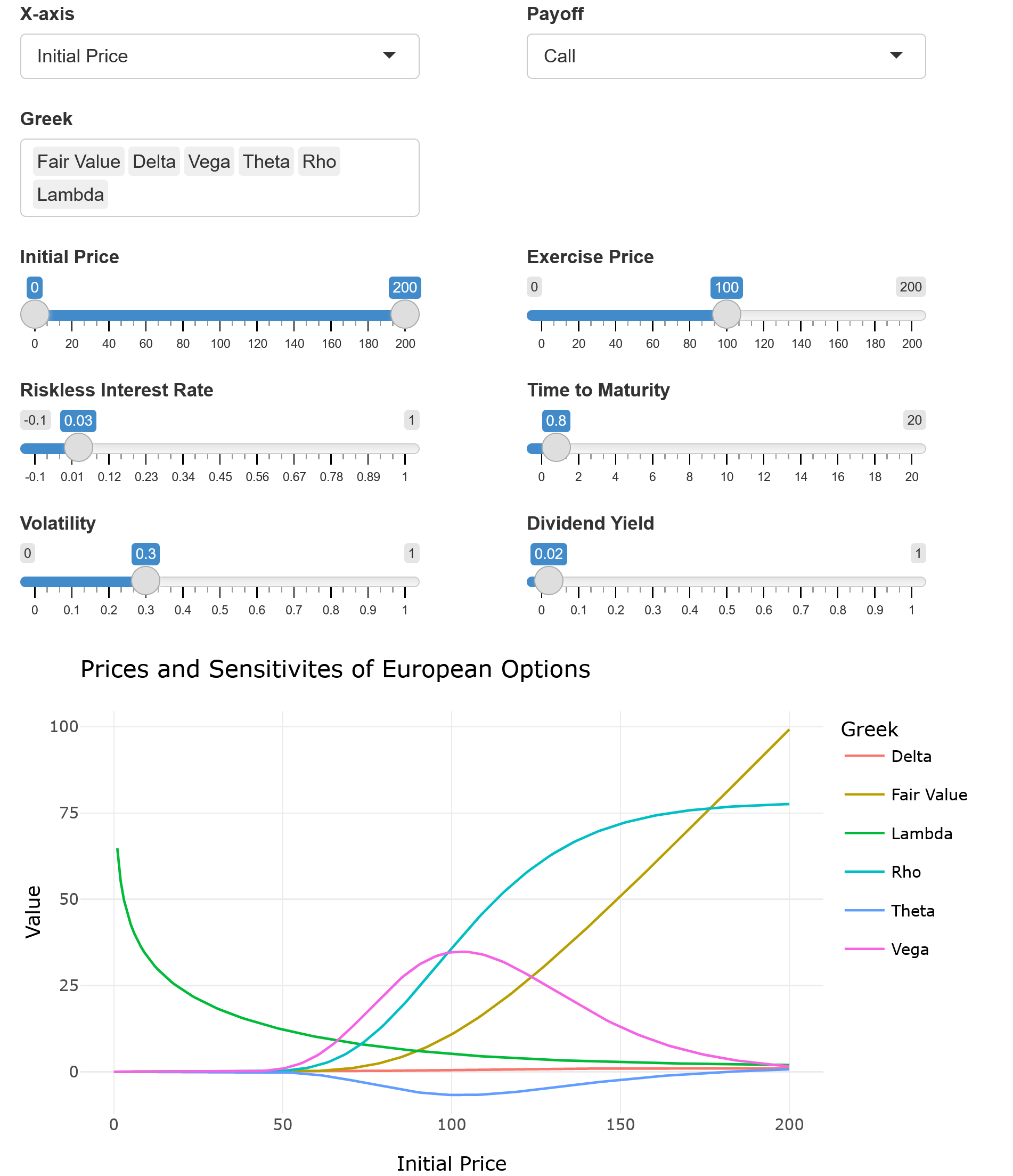# greeks: Sensitivities of Prices of Financial Options and Implied Volatilites

The package greeks provides functions to compute financial option prices and sensitivities of financial option prices for European, American, Asian, and Digital options in the Black Scholes model, and in more general jump diffusion models. Furthermore, based on the implementations of Vega, efficient functions for calculating implied volatilities not just for European options, but also for American, Asian, and digital options are provided.

Classical formulas are implemented for European options in the Black Scholes Model. Furthermore, functions to calculate Malliavin Monte Carlo Greeks are given, as presented e.g., in Hudde, A. & Rüschendorf, L. (2016). European and Asian Greeks for exponential Lévy processes (https://arxiv.org/abs/1603.00920). These functions work for classical payoff functions, as well as for any custom square integrable function provided by the user. Additionally, these calculations are not restricted to the Black Scholes model, but work for more general Lévy Jump diffusion model, which is also customizable by the user.

## Computing option prices and Greeks in the shiny app

The option prices and volatilities for European options can be displayed with the interactive shiny app by calling Greeks_UIGreeks_UI

## The function Greeks

Most of the options prices and Greeks can easily be calculated with the function Greeks.

# Load package

library(greeks)

# Option price and most common Greeks of a European call option on a share with
# price 100 and volatility of 30%, where the exercise price is 120, the time to
# maturity of 5 years, and the riskless interest rate of 1%.

Greeks(initial_price = 100,
exercise_price = 120,
r = 0.01,
time_to_maturity = 5,
volatility = 0.30,
payoff = "call")

##    fair_value         delta          vega         theta           rho
##  21.577149923   0.554941778  88.358901748  -2.989937331 169.585139380
##         gamma
##   0.005890593

# Option price and most common Greeks of an American put option on a share with
# price 100 and volatility of 25%, where the exercise price is 100, the time to
# maturity of 1 year, and the riskless interest rate of -0.5%.

Greeks(initial_price = 100,
exercise_price = 100,
r = -0.005,
time_to_maturity = 1,
volatility = 0.30,
payoff = "put",
option_type = "American")

##  fair_value       delta        vega       theta         rho       gamma
##  12.2027075  -0.4469782  39.5313017  -6.2141979 -56.9005269  -0.1275472

## Computing implied volatilities

The package greeks also provides a function to compute implied volatilities for a wide range of option types and payoff functions:

# Implied volatility of an Asian call option with on an option price of 15, a
# share price of 100, an exercise_price of 100, a risk-free interest rate of
# 0.05 and a time to maturity of 1.

Implied_Volatility(option_price = 15, initial_price = 100,
exercise_price = 100, r = 0.05, time_to_maturity = 1,
option_type = "Asian", payoff = "call")

##  0.6330451